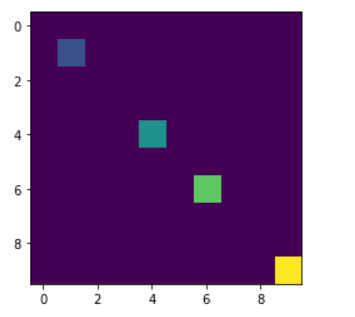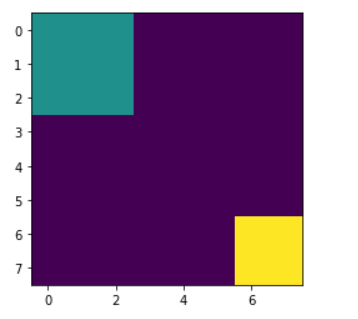Related Articles

# Mahotas – Sizes of Labelled Region

• Last Updated : 08 Jul, 2021

In this article we will see how we can obtain sizes of labelled region in mahotas. Labelled images are integer images where the values correspond to different regions. I.e., region 1 is all of the pixels which have value 1, region two is the pixels with value 2, and so on. By convention, region 0 is the background and often handled differently. We can create a labelled region with the help of mahotas.label method.
In order to do this we will use mahotas.label_size method

Syntax : mahotas.labelled_size(labelled_region)
Argument : It takes numpy.ndarray object as argument i.e labelled region
Return : It returns list of integer

Example 1:

## Python3

 `# importing required libraries``import` `mahotas as mh``import` `numpy as np``from` `pylab ``import` `imshow, show` `# creating region``# numpy.ndarray``regions ``=` `np.zeros((``10``, ``10``), ``bool``)` `# setting 1 value in the region``regions[``1``, ``1``] ``=` `1``regions[``6``, ``6``] ``=` `1``regions[``4``, ``4``] ``=` `1``regions[``9``, ``9``] ``=` `1` `# getting labelled function``labelled, nr_objects ``=` `mh.label(regions)` `# showing the image with interpolation = 'nearest'``imshow(labelled, interpolation ``=``'nearest'``)``show()` `# getting sizes of labelled region``sizes ``=` `mh.labelled.labelled_size(labelled)` `# printing sizes``for` `i ``in` `range``(``len``(sizes)):``               ` `    ``print``(``"Size of region "` `+` `str``(i) ``+` `" : "` `+` `str``(sizes[i]))``   `

Output :```Size of region 0 : 96
Size of region 1 : 1
Size of region 2 : 1
Size of region 3 : 1
Size of region 4 : 1```

Example 2:

## Python3

 `# importing required libraries``import` `mahotas as mh``import` `numpy as np``from` `pylab ``import` `imshow, show` `# creating region``# numpy.ndarray``regions ``=` `np.zeros((``10``, ``10``), ``bool``)` `# setting 1 value to the region``regions[:``3``, :``3``] ``=` `1``regions[``6``:, ``6``:] ``=` `1` `# getting labelled function``labelled, nr_objects ``=` `mh.label(regions)` `# showing the image with interpolation = 'nearest'``imshow(labelled, interpolation ``=``'nearest'``)``show()` `# getting sizes of labelled region``sizes ``=` `mh.labelled.labelled_size(labelled)` `# printing sizes``for` `i ``in` `range``(``len``(sizes)):``               ` `    ``print``(``"Size of region "` `+` `str``(i) ``+` `" : "` `+` `str``(sizes[i]))``   `

Output :```Size of region 0 : 75
Size of region 1 : 9
Size of region 2 : 16```

Attention geek! Strengthen your foundations with the Python Programming Foundation Course and learn the basics.

To begin with, your interview preparations Enhance your Data Structures concepts with the Python DS Course. And to begin with your Machine Learning Journey, join the Machine Learning – Basic Level Course

My Personal Notes arrow_drop_up